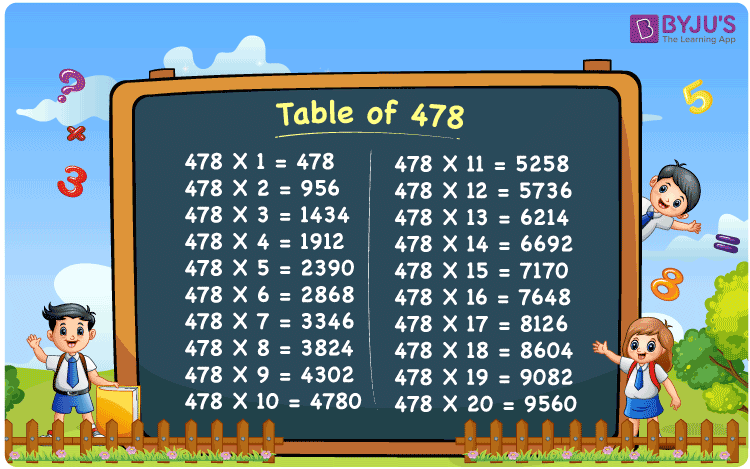Checkout JEE MAINS 2022 Question Paper Analysis : Checkout JEE MAINS 2022 Question Paper Analysis :

# Table of 478

The Table of 478 is presented here in table grid format to assist students in quickly checking the values of the multiples of 478. Solved examples are also available to assist with questions about the table of 478. Students can use a downloadable PDF of the multiplication table of 478 to memorise multiples of 478, and retain it for future reference.

## Table of 478 Chart## What is the 478 Times Table?

The 478 times table is the list of all multiples of 478. Any natural number multiplied by 478 is the multiple of 478. The multiplication table of 478 can be represented in both multiplication and addition form. The table below lists the multiples of 478 in both multiplication and addition form.

 478×1 = 478 478 478×2 = 956 478 + 478 = 956 478×3 = 1434 478 + 478 + 478 = 1434 478×4 = 1912 478 + 478 + 478 + 478 = 1912 478×5 = 2390 478 + 478 + 478 + 478 + 478 = 2390 478×6 = 2868 478 + 478 + 478 + 478 + 478 + 478 = 2868 478×7 = 3346 478 + 478 + 478 + 478 + 478 + 478 + 478 = 3346 478×8 = 3824 478 + 478 + 478 + 478 + 478 + 478 + 478 + 478 = 3824 478×9 = 4302 478 + 478 + 478 + 478 + 478 + 478 + 478 + 478 + 478 = 4302 478×10 = 4780 478 + 478 + 478 + 478 + 478 + 478 + 478 + 478 + 478 + 478 = 4780

## Multiplication Table of 478

The multiplication table of 478 is given below in tabular form:

 478 × 1 = 478 478 × 2 = 956 478 × 3 = 1434 478 × 4 = 1912 478 × 5 = 2390 478 × 6 = 2868 478 × 7 = 3346 478 × 8 = 3824 478 × 9 = 4302 478 × 10 = 4780 478 × 11 = 5258 478 × 12 = 5736 478 × 13 = 6214 478 × 14 = 6692 478 × 15 = 7170 478 × 16 = 7648 478 × 17 = 8126 478 × 18 = 8604 478 × 19 = 9082 478 × 20 = 9560

## Solved Examples on Table of 478

Q. What is the difference between the 18th multiple and the 20th multiple of 478?

Solution:

Using the multiplication table of 478

The 18th multiple of 478 = 8604

The 20th multiple of 478 = 9560

The difference between the 18th multiple and 20th multiple = 9560 – 8604 = 956

## Frequently Asked Questions on the Table of 478

### What is the table of 478?

The table of 478 is the list of all multiples of 478 starting from 478 × 1 = 478, 478 × 2 = 956, 478 × 3 = 1434, and so on.

### What is 5 times 478?

5 times 478 is 2390.

### Calculate the value of 478 times 10 minus 80.

478 times 10 minus 80 equals 2100.
I.e., 478 × 10 – 80 = 4700.